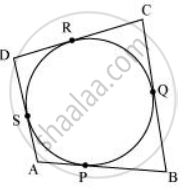# A Circle Touches All the Four Sides of a Quadrilateral Abcd. Prove that Ab + Cd = Bc + Da - Mathematics

A circle touches all the four sides of a quadrilateral ABCD. Prove that AB + CD = BC + DA

#### Solution

ABCD is a quadrilateral. Suppose a circle touches the sides AB, BC, CD and DA of the quadrilateral ABCD at P, Q, R and S, respectivelyWe know that the length of tangents drawn from an external point to a circle are equal.

DR = DS .....(1)

CR = CQ .....(2)

BP = BQ .....(3)

AP = AS .....(4)

Adding (1), (2), (3) and (4), we get

DR + CR + BP + AP = DS + CQ + BQ + AS

(DR + CR) + (BP + AP) = (DS + AS) + (CQ + BQ)

CD + AB = AD + BC

Or, AB + CD = BC + DA

Concept: Tangent to a Circle
Is there an error in this question or solution?